### Comprehensive & curriculum aligned

In this worksheet, students will add and subtract fractions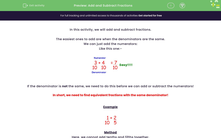Key stage:  KS 3

Curriculum topic:   Number

Curriculum subtopic:   Use Four Operations for All Numbers

Difficulty level:#### Worksheet Overview

In this activity, we will add and subtract fractions.

The easiest ones to add are when the denominators are the same.

We can just add the numerators:

Like this one:-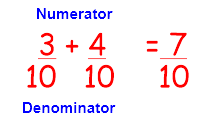Easy!!!!

If the denominator is not the same, we need to do this before we can add or subtract the numerators!

## In short, we need to find equivalent fractions with the same denominator!

Example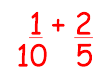Method

Here, we cannot add tenths and fifths together.

We look for the lowest common multiple of the denominators 10 and 5 = 10

The first one is already in tenths, so we need to multiply the second one by 2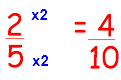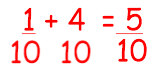We can even simplify again by dividing through by 5: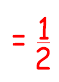Let's have a go at some questions now.### What is EdPlace?

We're your National Curriculum aligned online education content provider helping each child succeed in English, maths and science from year 1 to GCSE. With an EdPlace account you’ll be able to track and measure progress, helping each child achieve their best. We build confidence and attainment by personalising each child’s learning at a level that suits them.

Get started